Scalars: Scalars are mathematical entities which have only a magnitude­ (and no direction). Physical examples include mass and energy.
­
Vectors: Vectors are mathematical entities which have both a magnitude and a direction. Note that the location of the vector (for example, on which point a specific vector force is acting, or where a car with a given vector velocity is located) is not part of the vector itself. Physical examples include forces, velocities, momenta and locations. Note that as we show below, not all entities having a magnitude and direction are necessarily vectors (as is elaborated below).

We can also define the following:

Scalar functions: The scalar function (aka a scalar field) is a function which returns a scalar at each location of space. Examples include the pressure field (i.e., the pressure at each point in your room), the energy density in an electric field, etc.

Vector functions: The vector function (aka a vector field) is a function which returns a vector at each location of space. Examples include the gravitational field (at each location we have a different vector defining the local acceleration of gravity), the electric field, the velocity field of a flow (e.g., the speed of the water in your bathtub, which depends on its location relative to the sinkhole, etc.)

Notation:
• Scalar: Plain (or often italicized) letters: $a, k$, etc.
• Vectors: Either bold letters: $\bf{x},\bf{v}$ (often used in books), or a small vector sign: $\vec{x}$, or a small underline: $\underline{x}$. The latter two are the two standard hand written notation (how does one write bold letters with a pencil?). Some prefer the small arrow mark, while others prefer the underline. Do whatever you like, but be consistent!
• Magnitude of a vector: $A \equiv \left| {\bf A} \right|$.
• Unit vector in the direction of A (a vector which has a unit magnitude and the direction of A): $\hat{\bf A}$. Clearly, one has that $\left| \hat{\bf A} \right| = 1$ and ${\bf A}= \hat{\bf A} \left| {\bf A}\right|$.
• Position vector (aka radius vector or location vector): The vector ${\bf r}$ is the vector connecting the origin (usually denoted as $O$) to the location $P$ of a given point.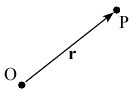The sum of two vectors is defined as the diagonal of the parallelogram formed when the two vectors ${\bf A}$ and ${\bf B}$ are placed at the same point, as is described in the diagram. Evidently, we see that the addition is commutative, that is, the order is not important: ${\bf A +B=B+A}$.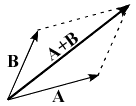The negative of a vector:

The vector $-{\bf A}$ is defined to be the same as vector ${\bf A}$, but with the opposite direction.  Thus, when we add a vector and its negative we obtained that ${\bf A} + ({\bf -A}) = 0$, as evident from the diagram.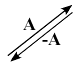Vector subtraction:

Vector subtraction ${\bf A - B}$ is defined as: ${\bf A + (-B)}$, that is, adding the negative of the subtracted vector ${\bf B}$, as is apparent in the figure.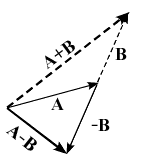Vector distributivity:

The graphical definition of vector addition also implies a very important characteristic: Vector addition is distributive, that is ${\bf A+ (B+C)=(A+B)+C}$. In other words, when we add three vectors, it does not matter whether we add the first pair first, or the second pair first. This is demonstrated in the following figure.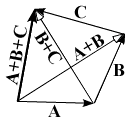Multiplication by a scaler:

Multiplying a vector by a scalar gives a new vector with the same direction but with a magnitude which is multiplied by the scaler. A negative scalar would yield a vector in the opposite direction as the original vector. For example: $(-1){\bf A} = - {\bf A}$.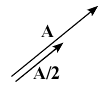Examples of Vectors:

The following are examples of vectors, that is, entities which have both a magnitude and a direction, and which can be added following the parallelogram rule:

1. Displacement vector (e.g., by how much a certain object moved) - the total displacement of an object satisfies the parallelogram definition of addition. If you first walk 1 mile east and then 1 mile north, you will end up at the same point as you would if you would first walk 1 mile north and then 1 mile east (assuming of course a flat earth!).
2. Forces are vectors (e.g., opposite forces cancel each other, two perpendicular forces add up to a force $\sqrt{2}$ larger and so forth).

Examples of non-vectors:

Just as a precaution, it is worth noting that not every mathematical entity which has a magnitude and a direction is necessarily a vector. Two interesting examples include:

• The polarization of light - As first glance, one may think that the polarization of light can be described by a vector, that is, a direction and a magnitude. However, this is not the case. Polarization (and therefore polarizers) have the characteristic that if you rotate them by 180°, they return to their original state (have you ever tried rotating polarizer sunglasses by 90° and 180°? If you didn't look for such glasses and try it). This is in contrast to vectors which become their negative when rotated by 180°, and it is only after a 360° rotation that they return to their original state. Hence, polarization cannot be described by a vector (for the curious: you actually can, though not in real space but in the Poincaré sphere instead. More about it here).
• Finite angle rotations - Rotations are another example of a mathematical entity which seems to be describable as a vector. The direction is the  axis of the rotation while the magnitude is the angle of rotation. However, it turns out (literally) that rotations are not commutative. That is, if you rotate by one rotation, and then by another, you do not end up in the same state as you would if you interchanged the order of the rotations. This is evident from the drawing below.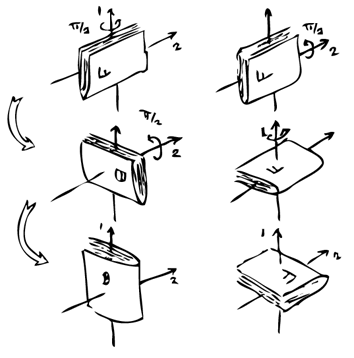Rotations are not commutative and therefore cannot be described by vectors: Rotating around axis 1 by π/2 (i.e., 90°) and then around axis 2, is not the same as the net rotation obtained when first rotating around axis 2 and then around axis 1. ­

## Blogroll

Sensible Climate Physics and more Other